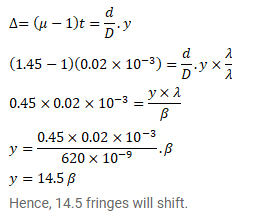# A transparent paper (refractive index=1.45) of thicknessQuestion:

A transparent paper (refractive index=1.45) of thickness $0.02 \mathrm{~mm}$ is pasted on one of the slits of a Young's slit experiment which uses monochromatic light of wavelength $620 \mathrm{~nm}$. How many fringes will cross through the centre if the paper is removed?

Solution: